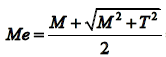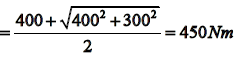Courses

# Torsion - MCQ Test 1

## 20 Questions MCQ Test GATE Mechanical (ME) 2022 Mock Test Series | Torsion - MCQ Test 1

Description
This mock test of Torsion - MCQ Test 1 for Mechanical Engineering helps you for every Mechanical Engineering entrance exam. This contains 20 Multiple Choice Questions for Mechanical Engineering Torsion - MCQ Test 1 (mcq) to study with solutions a complete question bank. The solved questions answers in this Torsion - MCQ Test 1 quiz give you a good mix of easy questions and tough questions. Mechanical Engineering students definitely take this Torsion - MCQ Test 1 exercise for a better result in the exam. You can find other Torsion - MCQ Test 1 extra questions, long questions & short questions for Mechanical Engineering on EduRev as well by searching above.
QUESTION: 1

### A solid circular shaft of 60 mm diameter transmits a torque of 1600 N.m. The value of maximum shear stress developed is:

Solution: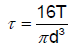QUESTION: 2

### The outside diameter of a hollow shaft is twice its inside diameter. The ratio of its torque carrying capacity to that of a solid shaft of the same material and the same outside diameter is:

Solution: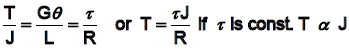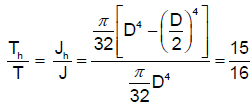QUESTION: 3

### A solid shaft transmits a torque T. The allowable shearing stress is τ.  What is the diameter of the shaft?

Solution:
QUESTION: 4

The diameter of a shaft is increased from 30 mm to 60 mm, all other conditions remaining unchanged. How many times is its torque carrying capacity increased?

Solution: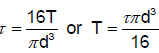for same material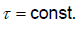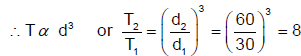QUESTION: 5

The magnitude of stress induced in a shaft due to applied torque varies

Solution:
QUESTION: 6

Maximum shear stress developed on the surface of a solid circular shaft under pure torsion is 240 MPa. If the shaft diameter is doubled then the maximum shear stress developed corresponding to the same torque will be:

Solution: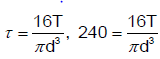if diameter doubled d 2d, then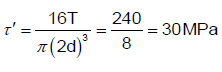QUESTION: 7

A torque T is applied at the free end of a stopped rod of circular cross-sections as shown in the figure. The shear modulus of the material of the rod is G. The expression for d to produce an angular twist θ at the free end is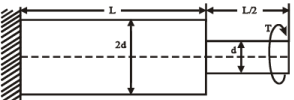Solution:

Angular twist at the free end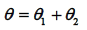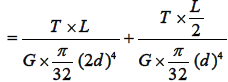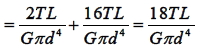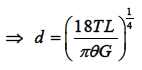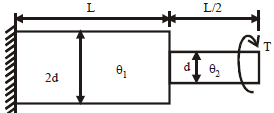QUESTION: 8

Maximum shear stress developed on the surface of a solid circular shaft under pure torsion is 240 MPa. If the shaft diameter is doubled, then what is the maximum shear stress developed corresponding to the same torque?

Solution:

Maximum shear stress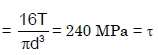Maximum shear stress developed when diameter is doubled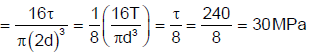QUESTION: 9

The magnitude of stress induced in a shaft due to applied torque varies

Solution:
QUESTION: 10

Assertion (A): In a composite shaft having two concentric shafts of different materials, the torque shared by each shaft is directly proportional to its polar moment of inertia.

Reason (R): In a composite shaft having concentric shafts of different materials, the angle of twist for each shaft depends upon its polar moment of inertia.

Solution:
QUESTION: 11

One-half length of 50 mm diameter steel rod is solid while the remaining half is hollow having a bore of 25 mm. The rod is subjected to equal and opposite torque at its ends. If the maximum shear stress in solid portion is τ or, the maximum shear stress in the hollow portion is:

Solution: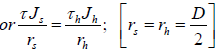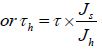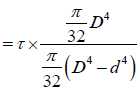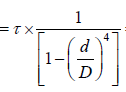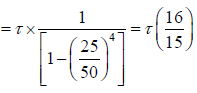QUESTION: 12

A shaft can safely transmit 90 kW while rotating at a given speed. If this shaft is replaced by a shaft of diameter double of the previous one and rotated at half the speed of the previous, the power that can be transmitted by the new shaft is:

Solution:
QUESTION: 13

A shaft is subjected to fluctuating loads for which the normal torque (T) and bending moment (M) are 1000 N-m and 500 N-m respectively. If the combined shock and fatigue factor for bending is 1.5 and combined shock and fatigue factor for torsion is 2, then the equivalent twisting moment for the shaft is:

Solution: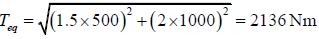QUESTION: 14

A shaft is subjected to simultaneous action of a torque T, bending moment M and an axial thrust F. Which one of the following statements is correct for this situation?

Solution:
QUESTION: 15

A circular shaft is subjected to the combined action of bending, twisting and direct axial loading. The maximum bending stress σ, maximum shearing force √3σ and a uniform axial stress σ(compressive) are produced. The maximum compressive normal stress produced in the shaft will be:

Solution:

Maximum normal stress = bending stress σ + axial stress (σ) = 2 σ
We have to take maximum bending stress σ is (compressive)

The maximum compressive normal stress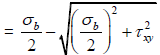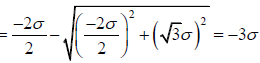QUESTION: 16

A hollow shaft of same cross-section area as solid shaft transmits

Solution:
QUESTION: 17

A solid shaft of diameter 100 mm, length 1000 mm is subjected to a twisting moment 'T‟ The maximum shear stress developed in the shaft is 60 N/mm2. A hole of 50 mm diameter is now drilled throughout the length of the shaft. To develop a maximum shear stress of 60 N/mm2 in the hollow shaft, the torque 'T‟ must be reduced by:

Solution: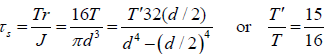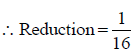QUESTION: 18

For the two shafts connected in parallel, find which statement is true?

Solution:
QUESTION: 19

Assertion (A): In theory of torsion, shearing strains increase radically away from the longitudinal axis of the bar.

Reason (R): Plane transverse sections before loading remain plane after the torque is applied.

Solution:
QUESTION: 20

A shaft is subjected to a bending moment M = 400 N.m alld torque T = 300 N.m The equivalent bending moment is:

Solution: